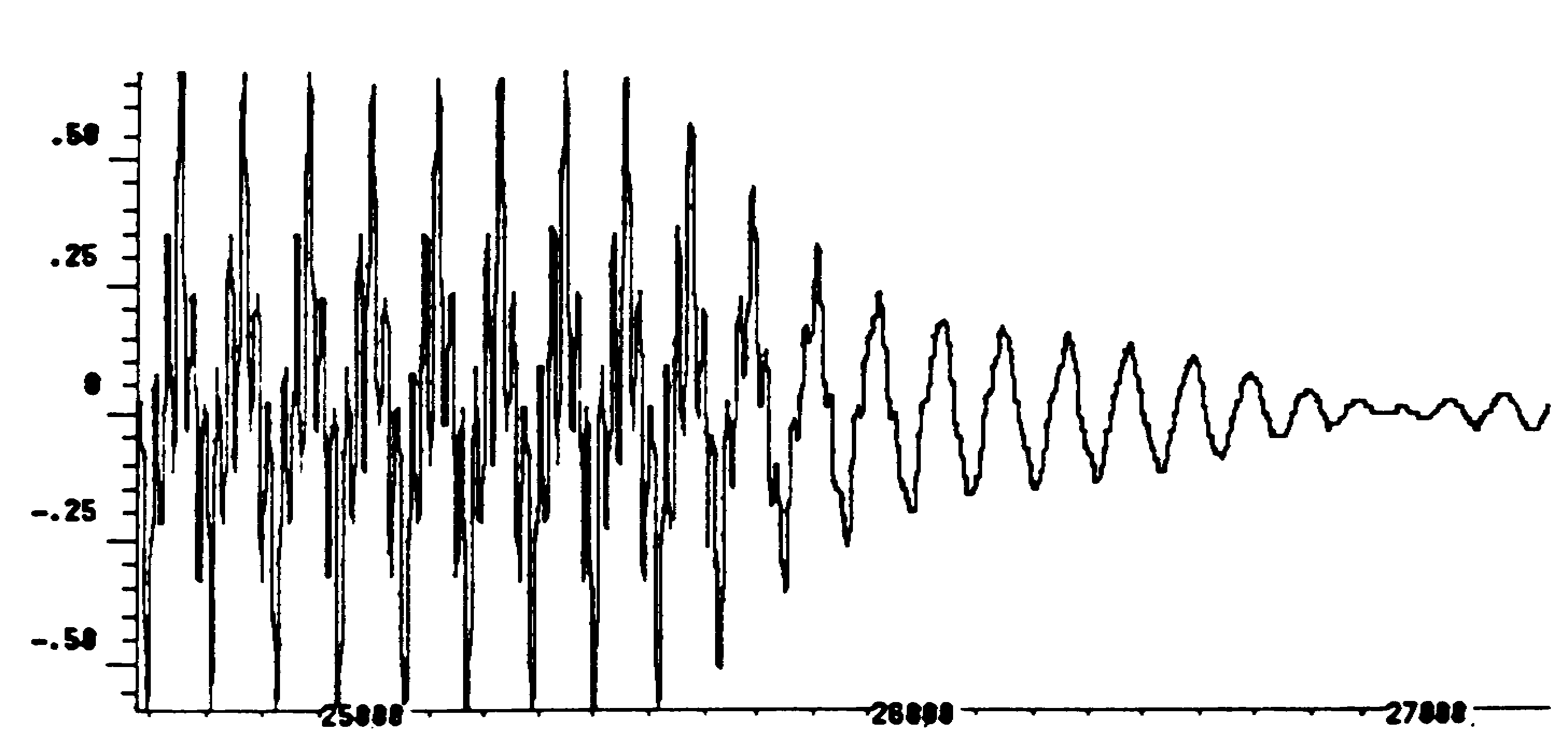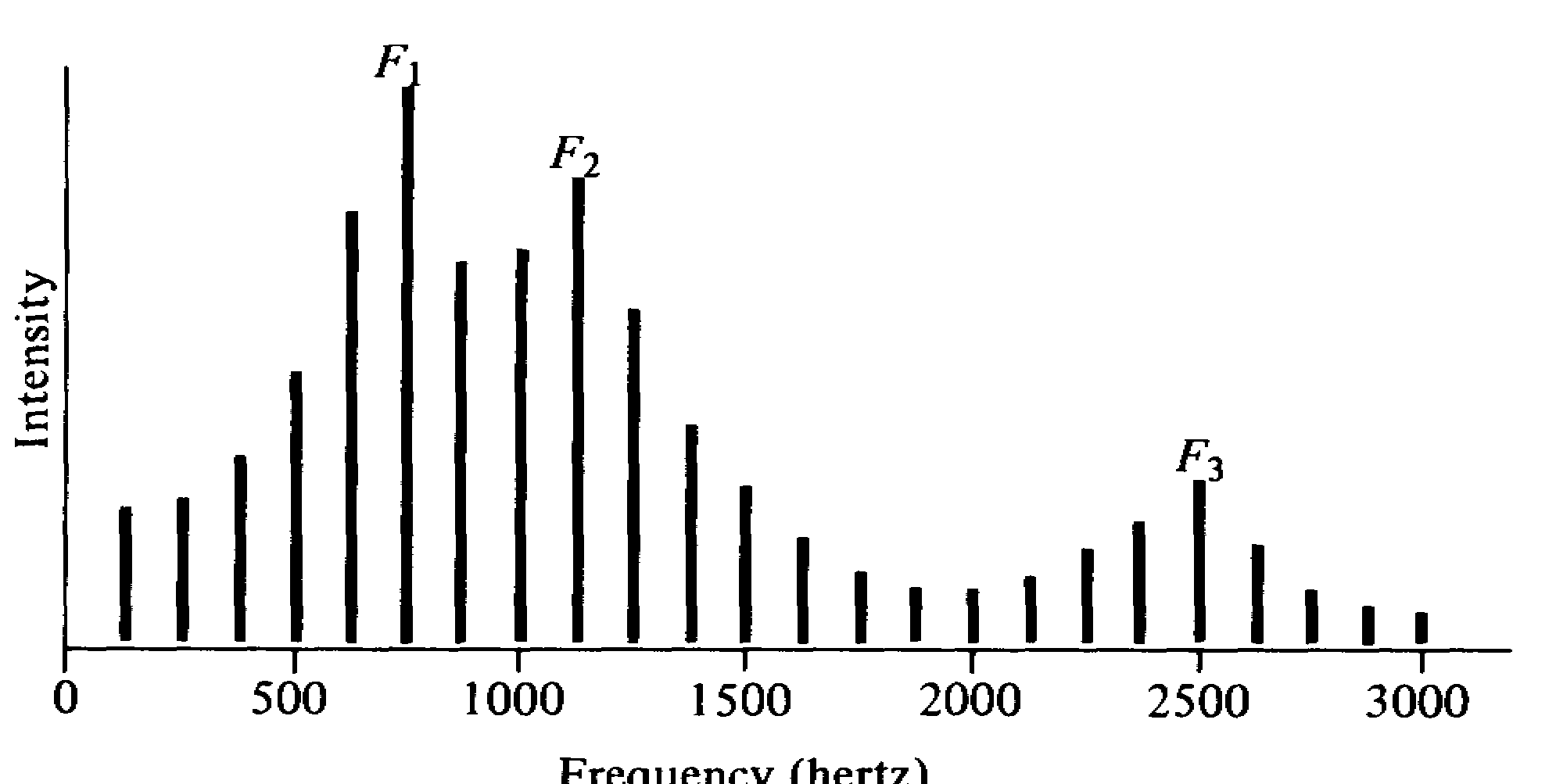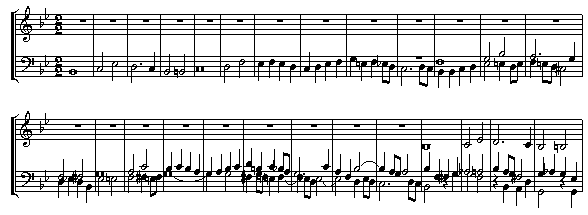MATH 254A

Time-frequency harmonic analysis

• A fundamental, and well-known, fact in harmonic analysis is that a function or signal f(t) can be analyzed both in physical space and in frequency space, with the two viewpoints  being connected by the Fourier transform.
• Here is an example of a function, described in physical space (the physical space variable  being time, measured in seconds):• And here is the same function, now described in frequency space (time-frequency being measured in Hertz.  Only intensity is shown; phase is suppressed):• It is slightly less well known that one can combine both the physical and frequency variables and analyze a function in time-frequency space, also known as the phase plane.  The precise  representation of functions on the phase plane is a little fuzzy, because of the Uncertainty Principle, however one can perform phase plane analysis rigorously and to good effect to problems in analysis.
• Actually, the idea of a phase space representation of sound waves predates that of a physical space or frequency space representation.  Here is an old example of a phase space representation:• In this course we shall develop some intuition for the phase plane, and then show how one can exploit this intuition to obtain results concerning
• Basic linear and multilinear estimates (Sobolev embedding, fractional Leibnitz rule, div-curl lemma, averaging lemma, etc.);
• Pseudo-differential operators;
• Propagation of singularities;
• Solvability of linear operators (the Levy example);
• Fourier summation; and the analysis of highly singular integrals (such as the bilinear Hilbert transform);
• Other topics as time permits.
• We will also show how such standard tools as Littlewood-Paley theory and wavelet decomposition can be viewed as ways to decompose the phase plane into manageable pieces.  We shall also study the Walsh phase plane, a discrete analogue of the Fourier phase plane.
• There is no formal prerequisite for this course, but it is recommended that you have already taken 245A or an equivalent course.  In particular you should be comfortable with the Fourier transform.
• There is no text; printed notes will be distributed during the course and will also be available on the web.  There are no examinations for this course, but homework will be assigned weekly, and will play a part in determining the final grade.